distancestree

## Introduction¶

Decision Trees are commonly described as being Classifiers(Regressors) that greedily search for the best division of the Input Space into exhaustive, mutually exclusive hyper-rectangles, returning as a prediction the most frequent class(mean value of the regressand) in the respective hyper-cube that a new example falls into. To make this somewhat technical explanation a little more tangible, let's plot a small example of a hypothetical data-generating process:

In :
import numpy as np
import matplotlib.pyplot as plt

np.random.seed(1234)

x1 = np.random.uniform(-5,5,1000)
x2 = np.random.uniform(-5,5,1000)
x1x2 = np.concatenate([x1.reshape(-1,1),x2.reshape(-1,1)],1)

xx, yy = np.meshgrid(np.arange(-5, 5, 0.01),
np.arange(-5, 5, 0.01))
point_space = np.c_[xx.ravel(), yy.ravel()]

y = np.zeros(1000)
y_space = np.zeros(len(point_space))

y[((x1<=2.5)&(x1>=-2.5))&((x2<=2.5)&(x2>=-2.5))] = 1

y_space[((point_space[:,0]<=2.5)&(point_space[:,0]>=-2.5))&(
(point_space[:,1]<=2.5)&(point_space[:,1]>=-2.5))] = 1

cols = np.array(["blue"]*1000)
cols[y==1] = "red"

plt.figure(figsize=(24, 12))
fig, (ax0, ax1) = plt.subplots(ncols=2, figsize=(12, 6))
ax0.set_title("Theoretic Class Boundaries")
ax0.contourf(xx, yy, y_space.reshape(xx.shape), cmap=plt.cm.bwr,alpha=0.3)
ax0.grid()

ax1.set_title("Sampled Data")
ax1.grid()
ax1.scatter(x1, x2, color = cols)
ax1.contourf(xx, yy, y_space.reshape(xx.shape), cmap=plt.cm.bwr,alpha=0.3)

plt.xlim(-5,5)
plt.ylim(-5,5)

Out:
(-5, 5)
<matplotlib.figure.Figure at 0x7f237ac5e750>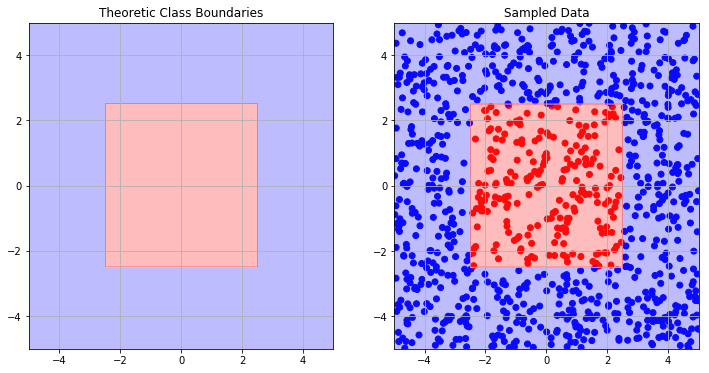The red class is obviously bounded by a rectangle in the two-dimensional space. To simplify things, there is no noise in this example and thus the class of sampled data is exactly determined by wether their coordinates lie within or outside the rectangle. A Decision Tree will search for the set of rectangles that minimizes the chance of misclassification of the training data - thus, we wish the predicted class boundaries to be as close as possible to the true class boundaries:

In :
from sklearn.tree import DecisionTreeClassifier

model = DecisionTreeClassifier().fit(x1x2, y)

pred = model.predict(point_space)
pred = pred.reshape(xx.shape)

plt.figure(figsize=(24, 12))
fig, (ax0, ax1) = plt.subplots(ncols=2, figsize=(12, 6))
ax0.set_title("Theoretic Class Boundaries")
ax0.contourf(xx, yy, y_space.reshape(xx.shape), cmap=plt.cm.bwr,alpha=0.3)
ax0.grid()

ax1.set_title("Predicted Class Boundaries")
ax1.contourf(xx, yy, pred, cmap=plt.cm.coolwarm,alpha=0.3)
ax1.grid()

<matplotlib.figure.Figure at 0x7f237adc38d0>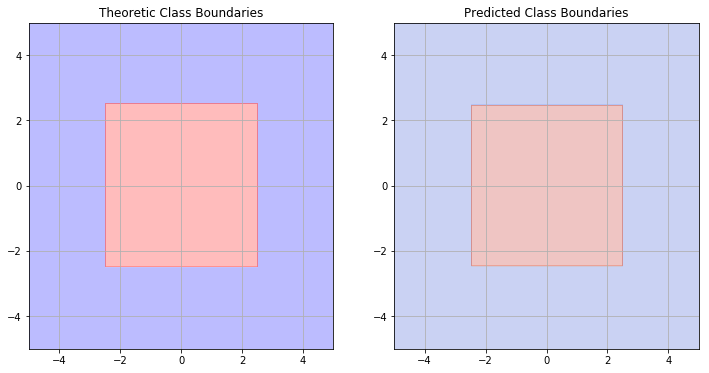The predicted class boundaries appear to match ground truth pretty well - probably not perfectly well, depending on the training samples. To achieve this precision, the Decision Tree needs a maximum depth of:

In :
model.tree_.max_depth

Out:
4

## Introducing non-rectangular data¶

In reality, the data generating process will hardly be as simple as above, so let's create another example with class boundary given by a circle centered at (0,0):

In :
y2 = np.zeros(1000)
y2_space = np.zeros(len(point_space))

y2[(x1**2+x2**2)<=5] = 1

y2_space[(point_space[:,0]**2+point_space[:,1]**2)<=5] = 1

cols2 = np.array(["blue"]*1000)
cols2[y2==1] = "red"

plt.figure(figsize=(24, 12))
fig, (ax0, ax1) = plt.subplots(ncols=2, figsize=(12, 6))
ax0.set_title("Theoretic Class Boundaries")
ax0.contourf(xx, yy, y2_space.reshape(xx.shape), cmap=plt.cm.bwr,alpha=0.3)
ax0.grid()

ax1.set_title("Sampled Data")
ax1.grid()
ax1.scatter(x1, x2, color = cols2)
ax1.contourf(xx, yy, y2_space.reshape(xx.shape), cmap=plt.cm.bwr,alpha=0.3)

plt.xlim(-5,5)
plt.ylim(-5,5)

Out:
(-5, 5)
<matplotlib.figure.Figure at 0x7f237aa41b10>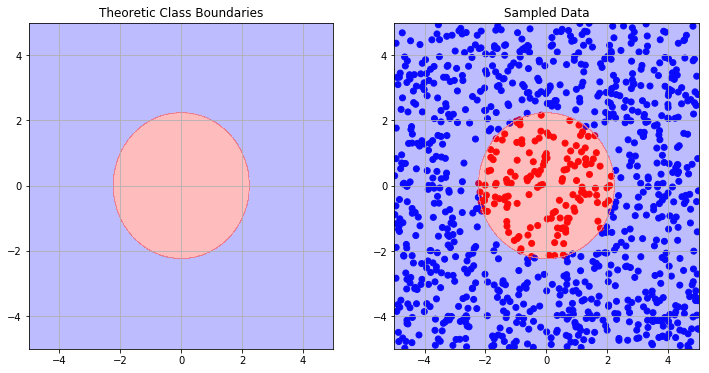As you might know we would need an infinite amount of rectangles becoming infinitesimally smaller the closer they are to the circle's closure to perfectly fill it. Adding that to the tiny area covered by the training data, it is a safe bet to assume that Decision Trees won't work well here:

In :
model2_1 = DecisionTreeClassifier().fit(x1x2, y2)

pred2_1 = model2_1.predict(point_space)

pred2_1 = pred2_1.reshape(xx.shape)

plt.figure(figsize=(24, 12))
fig, (ax0, ax1) = plt.subplots(ncols=2, figsize=(12, 6))
ax0.set_title("Theoretic Class Boundaries")
ax0.contourf(xx, yy, y2_space.reshape(xx.shape), cmap=plt.cm.bwr,alpha=0.3)
ax0.grid()

ax1.set_title("Predicted Class Boundaries")
ax1.contourf(xx, yy, pred2_1, cmap=plt.cm.coolwarm,alpha=0.3)
ax1.grid()

<matplotlib.figure.Figure at 0x7f237ac4f4d0>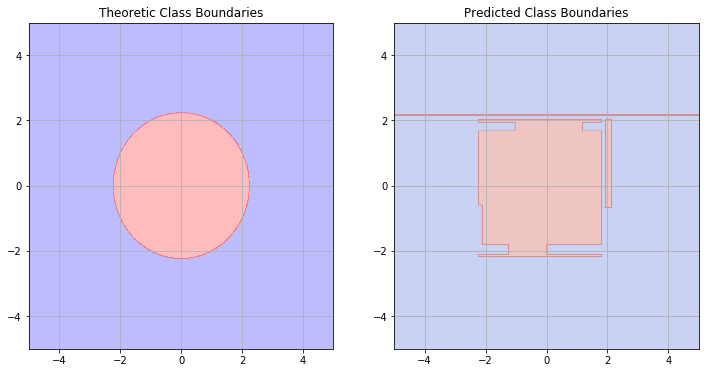In :
model2_1.tree_.max_depth

Out:
9

Despite having a deeper structure, the tree is unable to learn a good representation of the class boundaries.

## Fixing the issue¶

The obvious way out of the trouble is to just switch to another learning algorithm that is not restricted to rectangular class boundaries. However, we will most likely lose any model interpretability that we have with Decision Trees which, depending on the task, might be undesirable or even forbidden by law.

If you take a closer look at how the circular class-border is created above, you might see how a Decision Tree can learn a correct representation anyway:

In :
model2_2 = DecisionTreeClassifier().fit(np.concatenate([x1x2,(x1**2+x2**2).reshape(1000,1)],1), y2)

pred2_2 = model2_2.predict(np.concatenate([point_space,(point_space[:,0]**2+point_space[:,1]**2).reshape(
len(point_space),1)],1))

pred2_2 = pred2_2.reshape(xx.shape)

plt.figure(figsize=(24, 12))
fig, (ax0, ax1) = plt.subplots(ncols=2, figsize=(12, 6))
ax0.set_title("Theoretic Class Boundaries")
ax0.contourf(xx, yy, y2_space.reshape(xx.shape), cmap=plt.cm.bwr,alpha=0.3)
ax0.grid()

ax1.set_title("Predicted Class Boundaries")
ax1.contourf(xx, yy, pred2_2, cmap=plt.cm.coolwarm,alpha=0.3)
ax1.grid()

<matplotlib.figure.Figure at 0x7f23790bc110>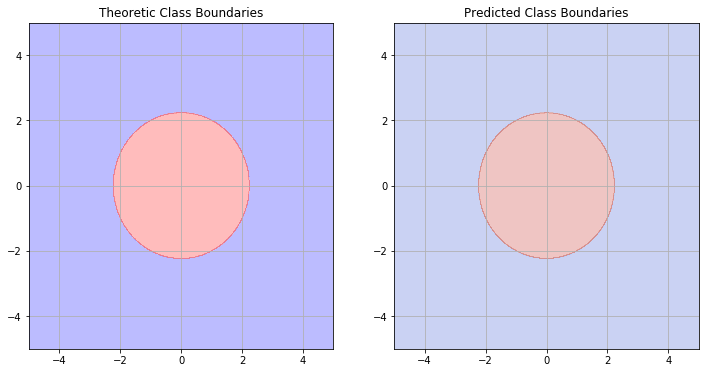In :
model2_2.tree_.max_depth

Out:
1

To fix the problem, I simply created another feature: $$X_3=X_1^2+X_2^2$$ which mimics the data-generating process. Adding $X_3$ to the training data, a plain Decision Tree is able to learn the class-border even easier (needing a more shallow structure) than the rectangular shape before. For real-world data, this example stresses the significant improvements that we can achieve through thoughtful feature engineering. In this toy-example this means the difference between using an easy-to-interpret white-box algorithm and a probable black-box solution. It is also worthwhile to look for new features that aren't as obvious as ratios or interaction-features (how often have you used the sum of two squared features as a new feature in your data?). One interesting Python package that generates new feature combinations is gplearn which can also be used for Symbolic Regression.

## Extending things to the real world¶

Despite the usefulness in the simulated data, we are unlikely to find data whose class-border is a perfect circle centered at the origin. Add noise to that and the chance becomes close to impossible. Below is another example (still without noise though), that I found far less 'perfect' than the ones before:

In :
y3 = np.zeros(1000)
y3_space = np.zeros(len(point_space))

y3[((x1+1)**2+(x2+1)**2)<=1.5] = 1
y3[((x1-1)**2+(x2-1)**2)<=1.5] = 1
y3[((x1+3)**2+(x2-2)**2)<=1.5] = 1
y3[((x1-2)**2+(x2+2.5)**2)<=1.5] = 1

y3_space[((point_space[:,0]+1)**2+(point_space[:,1]+1)**2)<=1.5] = 1
y3_space[((point_space[:,0]-1)**2+(point_space[:,1]+-1)**2)<=1.5] = 1
y3_space[((point_space[:,0]+3)**2+(point_space[:,1]-2)**2)<=1.5] = 1
y3_space[((point_space[:,0]-2)**2+(point_space[:,1]+2.5)**2)<=1.5] = 1

cols3 = np.array(["blue"]*1000)
cols3[y3==1] = "red"

plt.figure(figsize=(24, 12))
fig, (ax0, ax1) = plt.subplots(ncols=2, figsize=(12, 6))
ax0.set_title("Theoretic Class Boundaries")
ax0.contourf(xx, yy, y3_space.reshape(xx.shape), cmap=plt.cm.bwr,alpha=0.3)
ax0.grid()

ax1.set_title("Sampled Data")
ax1.grid()
ax1.scatter(x1, x2, color = cols3)
ax1.contourf(xx, yy, y3_space.reshape(xx.shape), cmap=plt.cm.bwr,alpha=0.3)

plt.xlim(-5,5)
plt.ylim(-5,5)

Out:
(-5, 5)
<matplotlib.figure.Figure at 0x7f237ae909d0>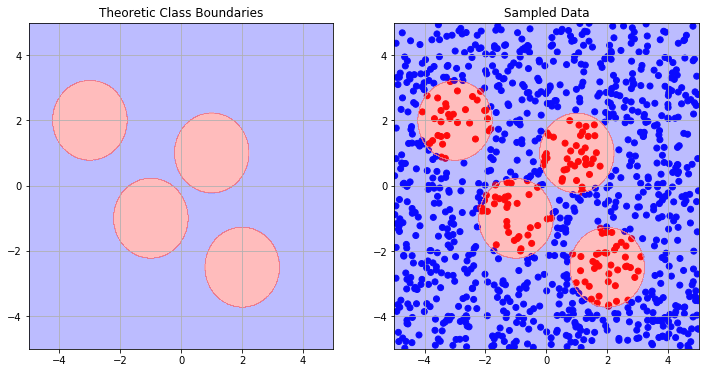As you can guess by now, I still wanted to solve this with a single Decision Tree of readable depth and, again, another feature transformation achieved the desired result - although not as nice as before. The sum-of-two-squared-features solution might look feasible, but in the real world the data dimensionality is probably too high to see this through graphical analysis. So what else can we do?

If you think back to geometry classes, you might remember that some points $x,y,z,...\in\mathbb{R}^k$ lying on the closure of a circle all have the same distance to the center of that circle, if the underlying space $\mathit{S}$ is Euclidean or is equipped with a Euclidean Norm respectively: $$||x||_2=\sqrt{x_1^2+x_2^2+...+x_k^2}$$ which also induces the distance between two points $x, y \in \mathit{S}$: $$d(x,y)=||x-y||_2$$ Now, if we knew the centers of each circle we could classify each point $y$ as "red" if its distance to any of the four circle centers was below a certain value - this brings us back to a structure of if-then rules that can easily be learned by Decision Trees. For real-world data though we don't know the circle centers, so the only thing possible is to greedily try out each point as a possible circle center and check if we can find a circular-like class-boundary around it.

In practice we will completely drop the original features and replace them by the (euclidean) distance between the observation and each other observation in the data-set. Thus, we will use the distance matrix instead of the data-matrix - you can think of it as a new data-matrix where the features for each observation are the (euclidean) distances to each other observation available.

To predict new observations, we calculate the distances of those new data-points to each observation in the training data and then let the fitted model do the prediction. Loosely speaking, this is also how kernel-methods work in Machine Learning and, besides their mathematical elegance, they are very powerful tools.

In :
#For the contourplot I coarsened the grid, since the original grid had one million observations, which
#would have meant calculating the 1.000(number of training instances) x 1.000.000 distances
xx2, yy2 = np.meshgrid(np.arange(-5, 5, 0.1),
np.arange(-5, 5, 0.1))
point_space2 = np.c_[xx2.ravel(), yy2.ravel()]

from scipy.spatial.distance import euclidean, pdist, squareform

#for model-training we can use the euclidean distance matrix
distances_train = squareform(pdist(x1x2))

distances_contour = np.zeros((len(point_space2),len(x1x2)))

#There is probably a more efficient way to do this through some matrix-multiplications instead of
#nested for-looping:
for i in range(len(point_space2)):
for j in range(len(x1x2)):
distances_contour[i,j] = euclidean(point_space2[i,:],x1x2[j,:])

In :
#each matrix has 1000 features - the euclidean distances to each observation in the 'training' data
print distances_train.shape
print distances_contour.shape

(1000, 1000)
(10000, 1000)

In :
model3_1 = DecisionTreeClassifier().fit(x1x2, y3)
pred3_1 = model3_1.predict(point_space)
pred3_1 = pred3_1.reshape(xx.shape)

model3_2 = DecisionTreeClassifier(max_depth=4).fit(distances_train, y3)
pred3_2 = model3_2.predict(distances_contour)

pred3_2 = pred3_2.reshape(xx2.shape)

fig, axes = plt.subplots(2, 2, figsize=(12, 12))
(ax0, ax1) = axes
(ax2, ax3) = axes
ax0.set_title("Theoretic Class Boundaries")
ax0.contourf(xx, yy, y3_space.reshape(xx.shape), cmap=plt.cm.bwr,alpha=0.3)
ax0.grid()

ax1.set_title("Predicted Class Boundaries - normal features")
ax1.contourf(xx, yy, pred3_1, cmap=plt.cm.coolwarm,alpha=0.3)
ax1.grid()

ax2.set_title("Predicted Class Boundaries - distance features")
ax2.contourf(xx2, yy2, pred3_2, cmap=plt.cm.coolwarm,alpha=0.3)
ax2.grid()

ax3.set_title("Theoretic Class Boundaries")
ax3.contourf(xx, yy, y3_space.reshape(xx.shape), cmap=plt.cm.bwr,alpha=0.3)
ax3.grid()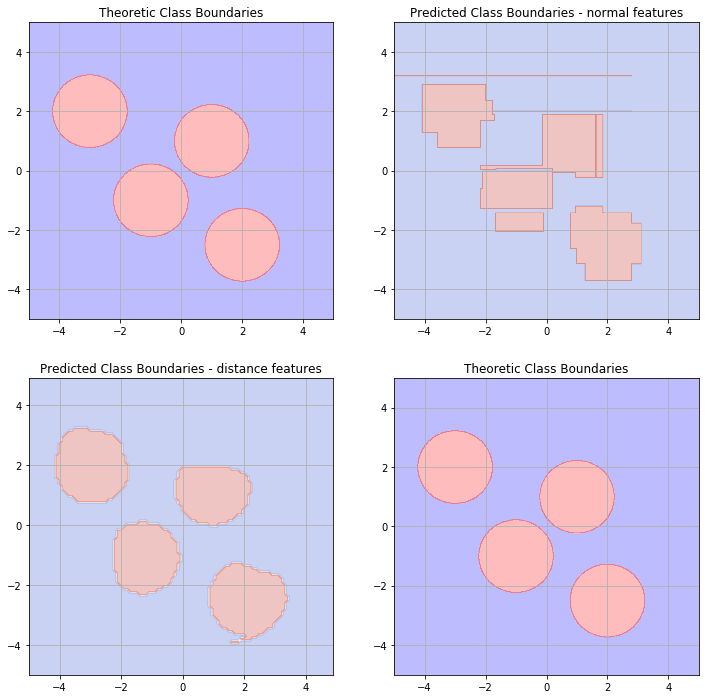In :
print model3_1.tree_.max_depth
print model3_2.tree_.max_depth

17
4


As I mentioned earlier, the resulting class-borders are not as perfect as before - this would only be the case if our trainining data contained the circles' centers and at least one point on the closure of each circle. According to measure theory, this has 0 probability of happening with finite training data available. Nevertheless, the Decision Tree constructed on the distance-features does a much better job than the one that was trained on the original features and is much more shallow. We can interpret each Decision Rule as $$if\,euclidean\,distance\,to\,X\leq some\,value,\,then\,...$$ I think this especially implies interesting possibilites for spatial analytics, making $X$ some kind of 'epicenter'. To get things even more realistic, the next step would be to discuss how to deal with non-continuous data where euclidean-distance is not a natural distance measure.
The major drawback of replacing the original features by the distance matrix is the loss of the big-data capability of Decision Trees, since calculating the distances becomes highly expensive with increasing amount of observations. Apart from that, the above example made me curious about training Decision Tree algorithms instead of Penalized Regression models on kernalized features which I will probably explore further in another post.